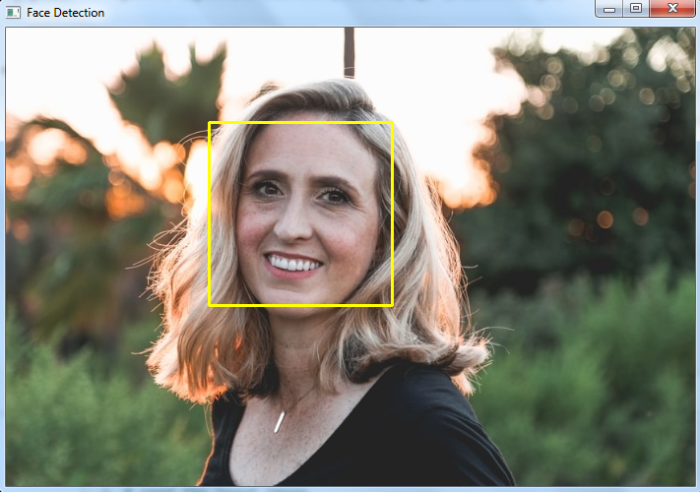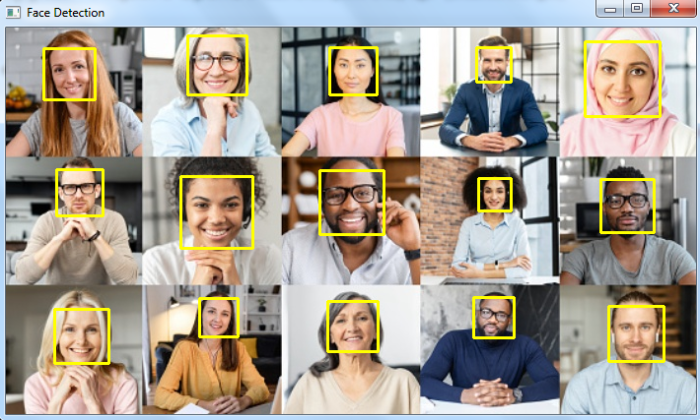# How to detect a face and draw a bounding box around it using OpenCV Python?

We detect a face in an image using a haar cascade classifier. A haar cascade classifier is an effective machine learning based approach for object detection. We can train our own haar cascade for training data but here we use already trained haar cascades for face detection.

We will use haarcascade_frontalface_alt.xml as a "haar cascade" XML file for face detection.

Note − Save all the haarcascade XML files in the haarcascades folder.

## Steps

We could follow the below steps to detect faces in an image and draw bounding boxes around them −

• Import the required library. In all the following examples, the required Python library is OpenCV. Make sure you have already installed it.

• Read the input image using cv2.imread(). Specify the full image path.

• Initiate a Haar cascade classifier object face_cascade = cv2.CascadeClassifier() for face detection. Pass the full path of the haar cascade xml file. You can use the haar cascade file haarcascade_frontalface_alt.xml to detect faces in the image.

• Detect faces in the input image using face_cascade.detectMultiScale(). It returns the coordinates of detected faces in (x,y,w,h) format.

• Draw the bounding rectangles around the detected faces in the original image using cv2.rectangle().

• Display the image with the drawn bounding rectangles around the faces.

Let's have a look at some examples for more clear understanding.

## Example

In this Python program, we detect a face and draw a bounding box around the detected face.

# import required library
import cv2

# convert to grayscale of each frames
gray = cv2.cvtColor(img, cv2.COLOR_BGR2GRAY)

# detects faces in the input image
print('Number of detected faces:', len(faces))\

# loop over all the detected faces
for (x,y,w,h) in faces:

# To draw a rectangle around the detected face
cv2.rectangle(img,(x,y),(x+w,y+h),(0,255,255),2)

# Display an image in a window
cv2.imshow('Face Detection',img)
cv2.waitKey(0)
cv2.destroyAllWindows()


Consider the following image as the Input File for this program −## Output

When you run the above Python program, it will produce the following output window −

Number of detected faces: 1


And we get the following output window −The bounding box around the face is drawn with yellow color.

## Example

In this Python program, we detect faces and draw bounding boxes around the detected faces in the input image.

import cv2
img1 =cv2.cvtColor(img, cv2.COLOR_BGR2GRAY)
ret,thresh = cv2.threshold(img1,170,255,0)

# draw different shapes of ellipses
img = cv2.ellipse(img,(368,250),(100,40),30,0,180,(0,255,255),-1)
img = cv2.ellipse(img,(150,170),(86,45),-30,0,360,(0,255,0),-1)
img = cv2.ellipse(img,(578,250),(60,130),0,0,340,(0,0,255),-1)

# display the image with drawn ellipses
cv2.imshow("Ellipses", img)
cv2.waitKey(0)
cv2.destroyAllWindows()


We will use this image as the Input File for this program −## Output

When you run the above Python program, it will produce the following output window −

Number of detected faces: 15


And we get the following output window −The bounding boxes around the faces are drawn with yellow color.